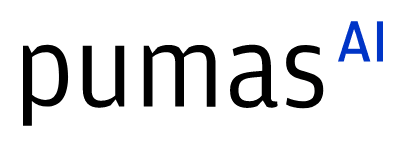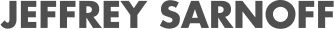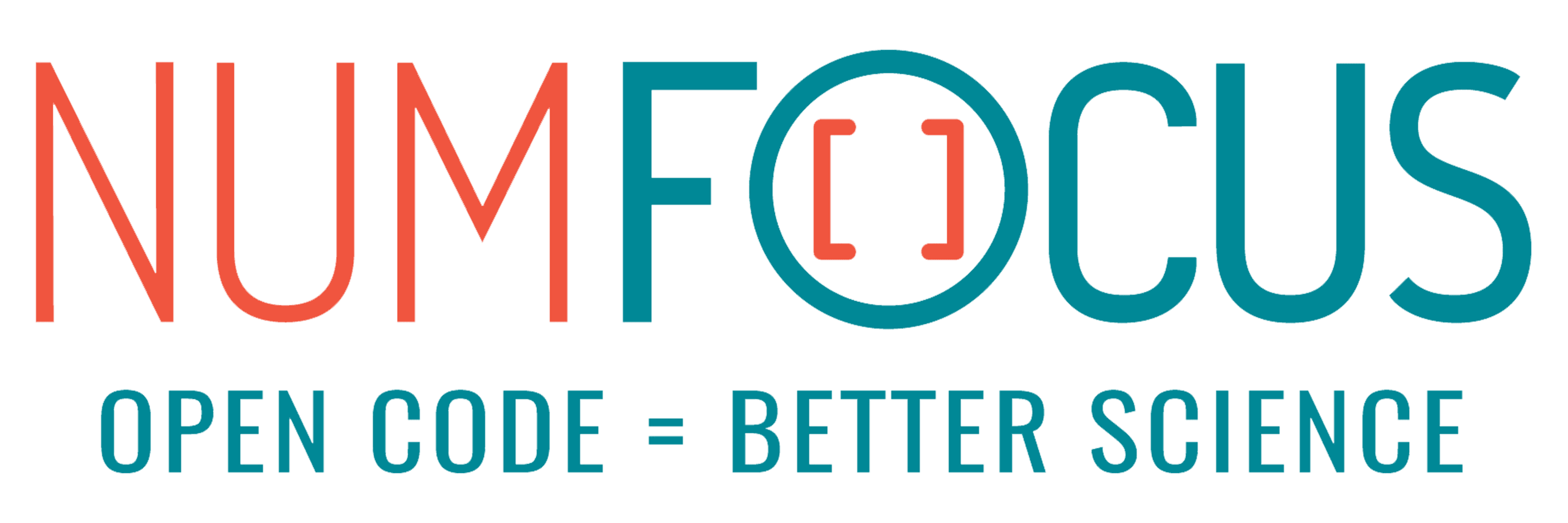# The role of (un)knowns in Julia's UDE modeling

07/28/2023, 3:20 PM3:30 PM UTC
32-D463 (Star)

#### Abstract:

In the context of the famous quote “It ain’t what you don’t know that gets you in trouble. It’s what you know for sure that just ain’t so” attributed to Mark Twain, we explore the magnitude of the trouble you get into when you introduce pathological assumptions in scientific machine modeling. Considering Universal Differential Equations we ask “what happens if our domain’s knowledge is incorrectly specified?” and answer showcasing the high interoperability of Julia.

#### Description:

One of the most promising evolutions in Machine Learning and AI is the approximation and analysis of differential equation systems with deep learning, i.e. Neural Network Differential Equations. Julia’s SciML ecosystem introduced an effective way to model natural phenomena as dynamical systems with Universal Differential Equations (UDE’s).The UDE framework enriches both classic and Neural Network differential equation modeling by combining an explicitly “known” term (which comes from our scientific knowledge of the natural phenomenon we are studying) with an “unknown” term (what rests to be discovered). Researchers are looking at how noise in the data observations, irregular observations, or inherent variability affect the performance of these techniques. This implicitly assumes that what we think about the natural phenomenon is correctly expressed in the known term. In this talk, instead, we are going to use Julia’s SciML ecosystem to study how an erroneous or partial understanding of a phenomenon (a perturbation of the known term) impacts our ability to discover the unknown component.

Within a UDE framework, the unknown terms, and therefore the overall functional forms of the dynamical system, are learned from the observational data by fitting a Neural Network. Methods such as sparse identification of non-linear dynamics (SINDy) can then be applied to simplify the fitted neural network and improve the performance of our model. We focus instead on the impact of possible pathologies in the design of a UDE system, and in particular, on possible errors we introduce in the expression of the known term. We pose the question, “what happens if our domain’s knowledge is incorrectly specified?”. In the context of the famous quote “It ain’t what you don’t know that gets you in trouble. It’s what you know for sure that just ain’t so” attributed to Mark Twain, we explore the magnitude of the trouble you get into.

### Mathematical Setting

More in detail, for a set of variables X, we consider a dynamical system of the form `dX/dt=F(X)=K(X)+U(X)`. In the following, we consider `K(X)` as known (ie.g., from domain knowledge), and `U(X)` as the “unknown” component we wish to discover. We sample observational data from `X(t)` at various points in time. Let `Kp(X)` be a perturbed version of K, that is, an erroneous specification of the domain knowledge. We try to recover `F(X)` from the data by optimizing a UDE of the form `dX/dt=Kp(X)+NN(X)`. For example, choosing `K(x)=sin(x)` we sample data from the system `dX/dt=sin(x)+exp(x)` and we try to recover it from a UDE such as `dX/dt=cos(x)+NN(X)`, where `Kp(x)=sin(x + π/2)` .

In this setting, we ask how strongly can we mispecify `K(X)` and still recover the functional form of `F(X)`?

We study the problem within the Julia’s SciML framework, exploiting its capacity of interoperating with symbolic computation systems, such as, Symbolics.jl and its high performance to explore a large space of original and perturbed functions.

### Future Development

The preliminary results raise interesting questions about the presence of undetectable domain knowledge errorse.

Our talk will interest both people who study UDEs for our cautionary and surprising results, and the wider audience interested more in the use of Julia in mathematical modeling for the encouraging examples of interoperability we present. The talk will present how Julia helped us in this experimental mathematical exercise, and offer many opportunities for further investigations.

The presentation will be as light as possible on the mathematical side, present ample examples of how the interoperability of Julia helped our analysis, and assumes little or no prior knowledge of UDE’s. Graphs and examples will also be used to aid understanding of the topic.